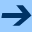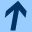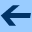Higher-order functions and function literals

# Higher-order functions and function literals

Here is a function that computes the sum of the integers from $$a$$ to $$b$$ (higher1.kts):

fun sumInt(a: Int, b: Int): Int {
var s = 0
for (i in a .. b)
s += i
return s
}


And here is code to compute the sum of the cubes of the integers from $$a$$ to $$b$$:

fun sumCubes(a: Int, b: Int): Int {
var s = 0
for (i in a .. b)
s += i * i * i
return s
}


Notice that sumInt and sumCubes are nearly identical—they only differ in the expression that is added to s in every iteration of the loop.

These two functions are special cases of computing the expression

$\sum_{i=a}^b f(i)$
for different choices of the function $$f$$.

If mathematics has a notation for this kind of common expression, then so should computer science. We should be able to write a function sum that takes the function $$f$$ as a parameter.

In Kotlin, we can do this as follows: (higher2.kts):

fun sum(a: Int, b: Int, f: (Int) -> Int): Int {
var s = 0
for (i in a..b)
s += f(i)
return s
}


Note the types of the three parameters: a and b are integers, but f has a more interesting type: (Int) -> Int. This type denotes a function from integers to integers. In general, the notation (A, B, ...) -> R denotes a function that takes arguments of types A, B, etc., and returns a result of type R.

To call the function sum, we need to provide an argument value for the parameter f. Modern programming languages like Kotlin (and Scala, Swift, Java since Java 8, and C++ since C++11) make it possible to define a function without having to give it a name. This is called a function literal, an anonymous function, or a lambda.

Note that for other basic objects, we define "nameless" objects all the time: We do not need to name every string or every integer. For instance, we don't have to write this:

>>> val str: String = "Hello CS109"
>>> val a: Int = 13
>>> println(str); println(a)


Instead, we simply write:

>>> println("Hello CS109"); println(13)


The value of an object written directly in the program is called a literal. For instance, writing 1234 is an integer literal, writing "CS109" is a string literal.

The syntax for function literals consists of braces containing the parameters, a right arrow, and some code to compute the result. For example, the function that raises an integer to its cube is written as

{ x: Int -> x * x * x }

A function literal that computes the sum of two integers looks like this:
{ a: Int, b: Int -> a + b }


The effect of executing a function literal is to create a function object (without giving it a name):

>>> { x: Int -> x * x * x }
kotlin.jvm.functions.Function1<java.lang.Integer, java.lang.Integer>


A function object exists on the heap, like every other object. We can assign a name to it, or store a reference to it in a collection or in another object. And, since it is a function object, we can call it like a function.

Here we create a function object and immediately call it with an argument:

>>> { x: Int -> x * x * x }(3)
27


Here we assign a name to the function object and use that to call it with different arguments:

s
>>> val f = { x: Int -> x * x * x }
>>> f(3)
27
>>> f(7)
343
>>> f(-30)
-27000


And here we store several function objects in a list:

>>> val g = listOf({ x: Int -> x * x },
...                { x: Int -> x * x * x },
...                { x: Int -> x * x * x * x })
>>> g(2)
4
>>> g(2)
8
>>> g(2)
16


Coming back to our function sum, we can now use it to compute the sum of integers and the sum of cubes:

>>> :load higher2.kts
>>> sum(1, 100, { x: Int -> x } )
5050
>>> sum(1, 100, { x: Int -> x * x * x } )
25502500


In fact, in this case the compiler can determine the type of the arguments in the function literal automatically. The compiler knows that the last argument of sum is a function of type (Int) -> Int, and so it knows that the type of any function literal written as an argument should be of this type. Therefore we can omit the type in the function literal:

>>> sum(1, 100, { x -> x } )
5050
>>> sum(1, 100, { x -> x * x * x } )
25502500


Furthermore, the tangle of closing parentheses at the end is a bit confusing, and so Kotlin has a nice convention: If the last argument in a function call is a function literal, we can write it after the closing parenthesis of the function call:

>>> sum(1, 100) { x -> x }
5050
>>> sum(1, 100) { x -> x * x * x }
25502500


Finally, another convention often simplifies the code: When a function literal has only one parameter, then we can omit the parameter and the right-arrow, and use the magic name it inside the function literal as the parameter: (higher3.kts):

>>> sum(1, 100) { it }
5050
>>> sum(1, 100) { it * it * it }
25502500


Functions like sum are called higher-order functions because they take another function object as an argument: A higher-order function is a "meta-function" that works on other functions.

Higher-order functions allow us to naturally express ideas where a function is part of the input to a problem, such as:

• print a table of function values for a given function (see table.kts)
• integrating a function numerically (see table.kts)
• finding a fixed point of a function.Higher-order functions and function literals